# MAFS.912.G-CO.3.9Archived StandardExport Print
Prove theorems about lines and angles; use theorems about lines and angles to solve problems. Theorems include: vertical angles are congruent; when a transversal crosses parallel lines, alternate interior angles are congruent and corresponding angles are congruent; points on a perpendicular bisector of a line segment are exactly those equidistant from the segment’s endpoints.
General Information
Subject Area: Mathematics
Domain-Subdomain: Geometry: Congruence
Cluster: Level 3: Strategic Thinking & Complex Reasoning
Cluster: Prove geometric theorems. (Geometry - Major Cluster) -

Clusters should not be sorted from Major to Supporting and then taught in that order. To do so would strip the coherence of the mathematical ideas and miss the opportunity to enhance the major work of the grade with the supporting clusters.

Date of Last Rating: 02/14
Status: State Board Approved - Archived
Assessed: Yes
Test Item Specifications

• Assessment Limits :
Items may assess relationships between vertical angles, special angles
formed by parallel lines and transversals, angle bisectors, congruent
supplements, congruent complements, and a perpendicular bisector
of a line segment.

Items may have multiple sets of lines and angles.

Items may include narrative proofs, flow-chart proofs, two-column
proofs, or informal proofs.

In items that require the student to justify, the student should not be
required to recall from memory the formal name of a theorem.

• Calculator :

Neutral

• Clarification :
Students will prove theorems about lines.

Students will prove theorems about angles.

Students will use theorems about lines to solve problems.

Students will use theorems about angles to solve problems.

• Stimulus Attributes :
Items may be set in a real-world or mathematical context.
• Response Attributes :
Items may require the student to give statements and/or
justifications to complete formal and informal proofs.

Items may require the student to justify a conclusion from a
construction.

Sample Test Items (2)
• Test Item #: Sample Item 1
• Question:

In the figure,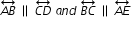. Let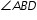measure (3x+4)º,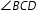measure(6x-8)º, and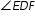measure (7x-20)º.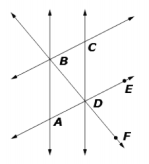Click on the blank to enter the degree measure that completes the equation shown.

• Difficulty: N/A
• Type: EE: Equation Editor

• Test Item #: Sample Item 2
• Question: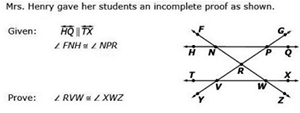Complete the proof by dragging the correct reasons to the table for line 3 and 6.

• Difficulty: N/A
• Type: DDHT: Drag-and-Drop Hot Text

## Related Courses

This benchmark is part of these courses.
1200400: Foundational Skills in Mathematics 9-12 (Specifically in versions: 2014 - 2015, 2015 - 2022, 2022 and beyond (current))
1206310: Geometry (Specifically in versions: 2014 - 2015, 2015 - 2022, 2022 and beyond (current))
1206320: Geometry Honors (Specifically in versions: 2014 - 2015, 2015 - 2022, 2022 and beyond (current))
1206315: Geometry for Credit Recovery (Specifically in versions: 2014 - 2015, 2015 - 2022, 2022 and beyond (current))
7912065: Access Geometry (Specifically in versions: 2015 - 2022, 2022 and beyond (current))

## Related Access Points

Alternate version of this benchmark for students with significant cognitive disabilities.

## Related Resources

Vetted resources educators can use to teach the concepts and skills in this benchmark.

## Formative Assessments

Proving the Corresponding Angles Theorem:

Students are asked to prove that corresponding angles formed by the intersection of two parallel lines and a transversal are congruent.

Type: Formative Assessment

Name That Triangle:

Students are asked to describe a triangle whose vertices are the endpoints of a segment and a point on the perpendicular bisector of a segment.

Type: Formative Assessment

Finding Angle Measures - 1:

Students are asked to find the measures of angles formed by three concurrent lines and to justify their answers.

Type: Formative Assessment

Finding Angle Measures - 4:

Students are asked to find the measure of an angle in a diagram containing two parallel lines and two transversals.

Type: Formative Assessment

Finding Angle Measures - 3:

Students are asked to find the measures of angles formed by two parallel lines and two transversals.

Type: Formative Assessment

Finding Angle Measures - 2:

Students are asked to find the measures of angles formed by two parallel lines and a transversal.

Type: Formative Assessment

Proving the Alternate Interior Angles Theorem:

In a diagram involving two parallel lines and a transversal, students are asked to use rigid motion to prove that alternate interior angles are congruent.

Type: Formative Assessment

Equidistant Points:

Students are asked to prove that a point on the perpendicular bisector of a line segment is equidistant from the endpoints of the segment.

Type: Formative Assessment

Proving the Vertical Angles Theorem:

Students are asked to identify a pair of vertical angles in a diagram and then prove that they are congruent.

Type: Formative Assessment

## Image/Photograph

Angles (Clipart ETC):

This large collection of clipart contains images of angles that can be freely used in lesson plans, worksheets, and presentations.

Type: Image/Photograph

## Lesson Plans

Engineering Design Challenge: Exploring Structures in High School Geometry :

Students explore ideas on how civil engineers use triangles when constructing bridges. Students will apply knowledge of congruent triangles to build and test their own bridges for stability.

Type: Lesson Plan

Parallel Thinking Debate:

Students prove theorems related to parallel lines using vertical, corresponding, and alternate interior angles.

Type: Lesson Plan

Vertical Angles: Proof and Problem-Solving:

In this lesson, students learn about the relationship between vertical angles by making inferences and proving the Vertical Angle Theorem. They then use this relationship to prove other angle relationships and to find angle measurements by using vertical angles.

Type: Lesson Plan

Proving and Using Congruence with Corresponding Angles:

Students, with the aid of GeoGebra software, will prove and use congruence of corresponding angles produced by parallel lines intersected by a traversal.

Type: Lesson Plan

Determination of the Optimal Point:

Students will use dynamic geometry software to determine the optimal location for a facility under a variety of scenarios. The experiments will suggest a relation between the optimal point and a common concept in geometry; in some cases, there will be a connection to a statistical concept. Algebra can be used to verify some of the conjectures.

Type: Lesson Plan

Parallel Lines:

Students will be able to prove that alternate interior angles are congruent and corresponding angles are congruent given two parallel lines and a traversal line. Students will use GeoGebra to explore real-world images to see if they can prove that their line segments are parallel.

Type: Lesson Plan

Location, Location, Location, Location?:

Students will use their knowledge of graphing concurrent segments in triangles to locate and identify which points of concurrency are associated by location with cities and counties within the Texas Triangle Mega-region.

Type: Lesson Plan

Accurately Acquired Angles:

Students will start the lesson by playing a game to review angle pairs formed by two lines cut by a transversal. Once students are comfortable with the angle pairs the teacher will review the relationships that are created once the pair of lines become parallel. The teacher will give an example of a proof using the angle pairs formed by two parallel lines cut by a transversal. The students are then challenged to prove their own theorem in groups of four. The class will then participate in a Stay and Stray to view the other group's proofs. The lesson is wrapped up through white board questions answered within groups and then as a whole class.

Type: Lesson Plan

Detemination of the Optimal Point:

Students will use dynamic geometry software to determine the optimal location for a facility under a variety of scenarios. The experiments will suggest a relation between the optimal point and a common concept in geometry; in some cases, there will be a connection to a statistical concept. Algebra can be used to verify some of the conjectures.

Type: Lesson Plan

Points equidistant from two points in the plane:

This task asks students to show how certain points on a plane are equidistant to points on a segment when placed on a perpendicular bisector.

Tangent Lines and the Radius of a Circle:

This problem solving task challenges students to find the perpendicular meeting point of a segment from the center of a circle and a tangent.

## Tutorials

Parallel lines and transversals:

In this tutorial, students will find the measures of angles formed when a transversal cuts two parallel lines.

Type: Tutorial

Parallel lines, transversals and triangles:

This tutorial shows students the eight angles formed when two parallel lines are cut by a transversal line. There is also a review of triangles in this video.

Type: Tutorial

Parallel lines and transversal lines:

Students will see in this tutorial the eight angles formed when two parallel lines are cut by a transversal line.

Type: Tutorial

Parallel lines and transversals:

In this tutorial, students will learn the angle measures when two parallel lines are cut by a transversal line.

Type: Tutorial

Sum of Exterior Angles of an Irregular Pentagon:

In this video, students will learn how to use what they know about the sum of angles in a triangle to determine the sum of the exterior angles of an irregular pentagon.

Type: Tutorial

Proving vertical angles are equal:

In this tutorial, students prove that vertical angles are equal. Students should have an understanding of supplementary angles before viewing this video.

Type: Tutorial

Finding the measure of vertical angles:

Students will use algebra to find the measure of vertical angles, or angles opposite each other when two lines cross. Students should have an understanding of complementary and supplementary angles before viewing this video.

Type: Tutorial

Introduction to vertical angles:

In this tutorial, students will use their knowledge of supplementary, adjacent, and vertical angles to solve problems involving the intersection of two lines.

Type: Tutorial

Using Algebra to Find Measures of Angles Formed from Transversal:

We will use algebra in order to find the measure of angles formed by a transversal.

Type: Tutorial

Figuring Out Angles Between Transversal and Parallel Lines:

We will be able to identify corresponding angles of parallel lines.

Type: Tutorial

Angles Formed by Parallel Lines and Transversals:

We will gain an understanding of how angles formed by transversals compare to each other.

Type: Tutorial

Proof: Vertical Angles are Equal:

This 5 minute video gives the proof that vertical angles are equal.

Type: Tutorial

## MFAS Formative Assessments

Equidistant Points:

Students are asked to prove that a point on the perpendicular bisector of a line segment is equidistant from the endpoints of the segment.

Finding Angle Measures - 1:

Students are asked to find the measures of angles formed by three concurrent lines and to justify their answers.

Finding Angle Measures - 2:

Students are asked to find the measures of angles formed by two parallel lines and a transversal.

Finding Angle Measures - 3:

Students are asked to find the measures of angles formed by two parallel lines and two transversals.

Name That Triangle:

Students are asked to describe a triangle whose vertices are the endpoints of a segment and a point on the perpendicular bisector of a segment.

Proving the Alternate Interior Angles Theorem:

In a diagram involving two parallel lines and a transversal, students are asked to use rigid motion to prove that alternate interior angles are congruent.

Proving the Vertical Angles Theorem:

Students are asked to identify a pair of vertical angles in a diagram and then prove that they are congruent.

## Student Resources

Vetted resources students can use to learn the concepts and skills in this benchmark.

Points equidistant from two points in the plane:

This task asks students to show how certain points on a plane are equidistant to points on a segment when placed on a perpendicular bisector.

Tangent Lines and the Radius of a Circle:

This problem solving task challenges students to find the perpendicular meeting point of a segment from the center of a circle and a tangent.

## Tutorials

Parallel lines and transversals:

In this tutorial, students will find the measures of angles formed when a transversal cuts two parallel lines.

Type: Tutorial

Parallel lines, transversals and triangles:

This tutorial shows students the eight angles formed when two parallel lines are cut by a transversal line. There is also a review of triangles in this video.

Type: Tutorial

Parallel lines and transversal lines:

Students will see in this tutorial the eight angles formed when two parallel lines are cut by a transversal line.

Type: Tutorial

Parallel lines and transversals:

In this tutorial, students will learn the angle measures when two parallel lines are cut by a transversal line.

Type: Tutorial

Sum of Exterior Angles of an Irregular Pentagon:

In this video, students will learn how to use what they know about the sum of angles in a triangle to determine the sum of the exterior angles of an irregular pentagon.

Type: Tutorial

Proving vertical angles are equal:

In this tutorial, students prove that vertical angles are equal. Students should have an understanding of supplementary angles before viewing this video.

Type: Tutorial

Finding the measure of vertical angles:

Students will use algebra to find the measure of vertical angles, or angles opposite each other when two lines cross. Students should have an understanding of complementary and supplementary angles before viewing this video.

Type: Tutorial

Introduction to vertical angles:

In this tutorial, students will use their knowledge of supplementary, adjacent, and vertical angles to solve problems involving the intersection of two lines.

Type: Tutorial

Using Algebra to Find Measures of Angles Formed from Transversal:

We will use algebra in order to find the measure of angles formed by a transversal.

Type: Tutorial

Figuring Out Angles Between Transversal and Parallel Lines:

We will be able to identify corresponding angles of parallel lines.

Type: Tutorial

Angles Formed by Parallel Lines and Transversals:

We will gain an understanding of how angles formed by transversals compare to each other.

Type: Tutorial

Proof: Vertical Angles are Equal:

This 5 minute video gives the proof that vertical angles are equal.

Type: Tutorial

## Parent Resources

Vetted resources caregivers can use to help students learn the concepts and skills in this benchmark.